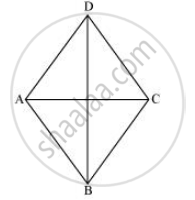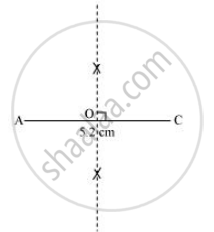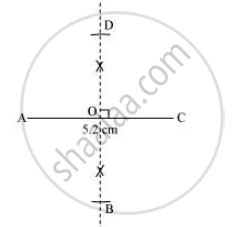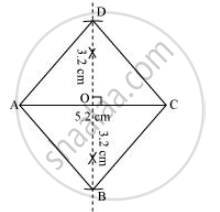# Draw the Following: a Rhombus Whose Diagonals Are 5.2 Cm and 6.4 Cm Long. - Mathematics

Draw the following:

A rhombus whose diagonals are 5.2 cm and 6.4 cm long.

#### Solution

In a rhombus, diagonals bisect each other at 90º. Therefore, the given rhombus ABCD can be drawn as follows.

1) A rough sketch of this rhombus ABCD is as follows.2) Draw a line segment AC of 5.2 cm and draw its perpendicular bisector. Let it intersect the line segment AC at point O.3) Draw arcs of "6.4 cm"/2 = 3.2 cm on both sides of this perpendicular bisector. Let the arcs intersect the perpendicular bisector at point B and D.4) Join points B and D with points A and C.ABCD is the required rhombus.

Is there an error in this question or solution?
Chapter 4: Practical Geometry - Exercise 4.5 [Page 68]

#### APPEARS IN

NCERT Class 8 Maths
Chapter 4 Practical Geometry
Exercise 4.5 | Q 2 | Page 68

Share